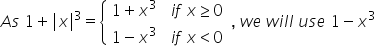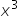Maths-
General
Easy

###Hint:

In this question we will useas the infinity is negative. Then we will divide the equation byand after simplifying we will put the value of x.

## Book A Free Demo+91